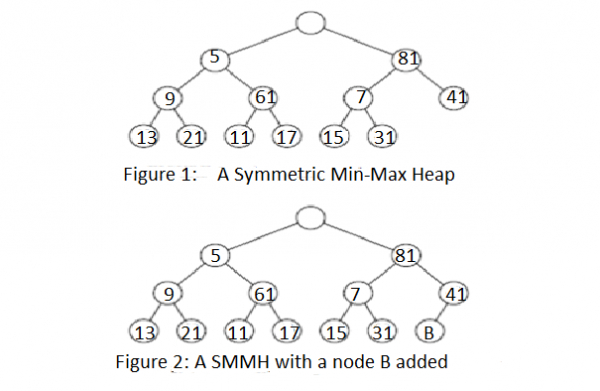# Symmetric Min-Max Heaps

Data Structure AlgorithmsAnalysis of AlgorithmsAlgorithms

A symmetric min-max heap (SMMH) is defined as a complete binary tree in which each node except the root has exactly one element. The root of an SMMH be empty and the total number of nodes in the SMMH is m + 1, where m is the number of elements.

Let y be any node of the SMMH. Let elements(y) be the elements in the sub tree rooted at y but excluding the element (if any) in y. Assume that elements(y) j= ∅. y satisﬁes the following properties:

• The left child of y has the minimum element in elements(y).
• The right child of y (if any) has the maximum element in elements(y).

Figure 1 shows an example SMMH that has 12 elements.

When y denotes the node with 81, elements(y) = {7, 15, 31, 41}; the left child of y has the minimum element 6 in elements(y); and the right child of y has the maximum element 41 in elements(y). We may verify that every node y of this SMMH satisﬁes the stated properties.

Since an SMMH is denoted as a complete binary tree, it is stored as an implicit data structure implementing the standard mapping of a complete binary tree into an array. When m = 1, the minimum and maximum elements are the same and are contained in the left child of the root of the SMMH.When m > 1, the minimum element is in the left child of the root and the maximum is in the right child of the root. So the getMin and getMax operations consume O(1) time.

It is easy to see that an m + 1-node complete binary tree with an empty root and one element in every other node is an SMMH iff the following are true −

A1 − For every node y that has a right sibling, the element in y is less than or equal to that in the right sibling of y.

A2 − For every node y that has a grandparent, the element in the left child of the grandparent is less than or equal to that in y.

A3 − For every node y that has a grandparent, the element in the right child of the grandparent is greater than or equal to that in y.

Notice that if property A1 is satisﬁed at node y, then at most one of A2 and A3 may be violated at y. Implementing properties A1 through A3 we reach at simple Algorithms to insert and remove elements. These Algorithms are simple adaptations of the corresponding Algorithms for min and max heaps. Their complexity is O(log m).

Here, we specify the insert operation. Suppose we wish to insert 3 into the SMMH of Figure 1. Since an SMMH is a complete binary tree, we must add a new node to the SMMH in the position shown in Figure 2; the new node is labelled as B.

In our example, B will denote an empty node. Now 3 is inserted at node B.